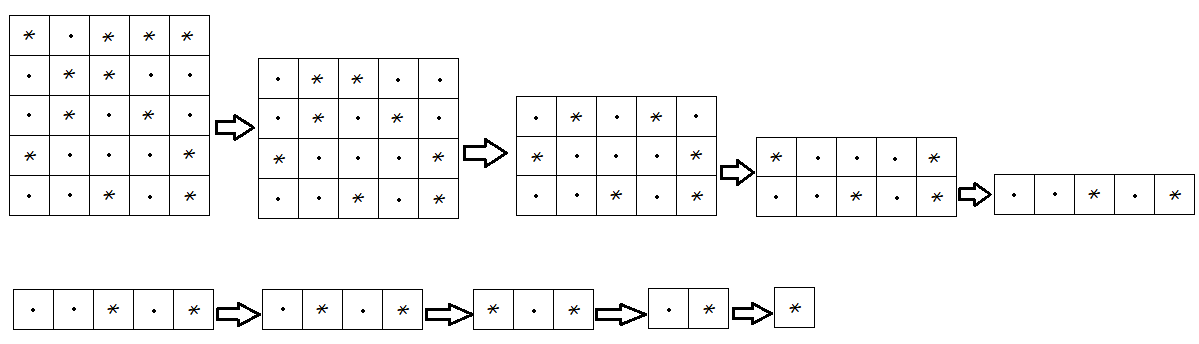# The Pattern

## Problem:-

Joey is always in fond of playing with patterns. So on his birthday his uncle gave him a mat.  Mat is a rectangular grid

$\left(N\ast M\right)$

. i.e. there are

$N$

rows and

$M$

columns drawn on the mat.  Each cell of this grid is filled with either dot

$\left(.\right)$

or star

$\left(\ast \right)$

. Now Joey starts to fold this mat.  Firstly he folds the mat along the rows (top to down) until it transforms into a

$\left(1\ast M\right)$

grid (Neglect width of mat on each fold). After that he starts folding this along the columns (left to right) and finally transforms into a single cell. At the end Joey wants to know what will be on the top of that cell

$\left($

dot

$\left(.\right)$

or star

$\left(\ast \right)\right)$

.

See the below image for more explanation.When star

$\left(\ast \right)$

come over dot

$\left(.\right)$

it converted to dot

$\left(.\right)$

When star

$\left(\ast \right)$

come over star

$\left(\ast \right)$

, it remains star

$\left(\ast \right)$

When dot

$\left(.\right)$

come over dot

$\left(.\right)$

, it remains dot

$\left(.\right)$

When dot

$\left(.\right)$

come over star

$\left(\ast \right)$

, it converted to star

$\left(\ast \right)$

Input:
First line of Input contains an integer

$T$

, denoting no of testcases.
First line of each testcase contains two integers

$N$

and

$M$

, no of rows and columns in the grid(respectively).
Each of next

$N$

lines contains a string of length

$M$

.

Output:
For each testcase print a single line, the character on the top of folded mat.
Constraints:

$1\le T\le 100$

$1\le N,M\le {10}^{3}$

SAMPLE INPUT

`15 5*.***.**...*.*.*...*..*.*`
SAMPLE OUTPUT

`*`
Time Limit:1.0 sec(s) for each input file.
Memory Limit:256 MB
Source Limit:1024 KB

## solution:-

#include<stdio.h>
void main()
{
int t,n,m;
char s;
scanf(“%d”,&t);
for(int i=0;i<t;i++)
{
scanf(“%d%d”,&n,&m);
for(int j=0;j<n;j++)
scanf(“%s”,s[j]);
printf(“%cn”,s[n1][m1]);
}
}